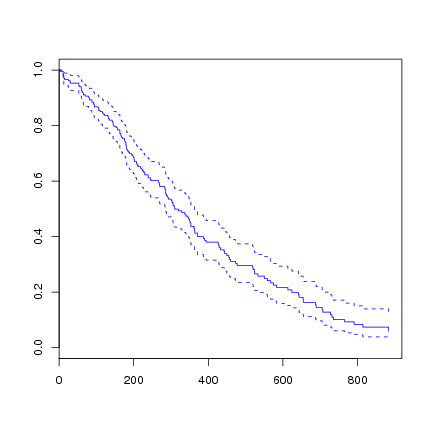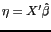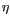DATA MINING
Desktop Survival Guide
by Graham Williams### Lung

We illustrate building a Cox proportional hazards model to the lung dataset.

 > library(survival) > l.coxph <- coxph(Surv(time, status) ~ sex, data=lung) > summary(l.coxph) 

 Call: coxph(formula = Surv(time, status) ~ sex, data = lung) n= 228 coef exp(coef) se(coef) z Pr(>|z|) sex -0.5310 0.5880 0.1672 -3.176 0.00149 ** --- Signif. codes: 0 ‘***’ 0.001 ‘**’ 0.01 ‘*’ 0.05 ‘.’ 0.1 ‘ ’ 1 exp(coef) exp(-coef) lower .95 upper .95 sex 0.588 1.701 0.4237 0.816 Rsquare= 0.046 (max possible= 0.999 ) Likelihood ratio test= 10.63 on 1 df, p=0.001111 Wald test = 10.09 on 1 df, p=0.001491 Score (logrank) test = 10.33 on 1 df, p=0.001312 

Here the coef is the estimated logarithm of the hazard ratio of the variable, sex in this case. A value of sex=1 is Male and sex=2 is Female. The hazard ratio is for the second group relative to the first group. In this case this is Female versus Male. So the coef -0.5310 is the estimated logarithm of the hazard ratio for Females versus Males.

Converting the log of the hazard ratio to the actual estimated hazard ratio we exp it: exp(coef). In this case exp(-0.5310) = 0.588. This estimated hazard ratio tells us that females have a lower risk of death (i.e., higher survival rate) than males. The exp(-coef) is 1/exp(coef), or the inverse ratio. Here it is exp(0.5310) = 1.700632. This says males have a higher risk of death (i.e., lower survival rate) than females.

We can plot the survival curve from the fitted Cox model.

 > plot(survfit(l.coxph), col=4)As usual, the predict function can be used to predict on new data. There are four types of predictions available.

 > results <- lung[c('time', 'status')] > results <- cbind(results, lp=predict(l.coxph, type="lp")) > results <- cbind(results, risk=predict(l.coxph, type="risk")) > results <- cbind(results, expected=predict(l.coxph, type="expected")) > results <- cbind(results, terms=predict(l.coxph, type="terms")) > l.coxph.age.results <- results > head(l.coxph.age.results) 

  time status lp risk expected sex 1 306 2 0.2096146 1.233203 0.8280030 0.2096146 2 455 2 0.2096146 1.233203 1.3880051 0.2096146 3 1010 1 0.2096146 1.233203 3.5060567 0.2096146 4 210 2 0.2096146 1.233203 0.5185439 0.2096146 5 883 2 0.2096146 1.233203 3.5060567 0.2096146 6 1022 1 0.2096146 1.233203 3.5060567 0.2096146 

With type="lp", the linear predictor is calculated (). Todo: Check: This is interpreted as the individual having a lower or higher risk than the (training) population, depending on whether the value is below zero or above zero. This is related to the point below that the data is zeroed, and is interpreted then as being lower or higher than the mean survival. We can deconstruct the calculation of this value:

 > coef(l.coxph) 

  sex -0.5310235 

 > predict(l.coxph, newdata=lung[1,], type="lp") 

  [,1] 1 0.2096146 

 > (lung[1,"age"] - l.coxph$means) %*% coef(l.coxph)["age"]    [,1] [1,] NA  This illustrates how the data is zeroed by subtracting the means. The means are calculated on the training data and used to zero on the new data. With type="risk", the risk for an individual relative to the average subject within the dataset is calculated ().  > predict(l.coxph, newdata=lung[1,], type="risk")    [,1] 1 1.233203   > exp((lung[1,"age"] - l.coxph$means) %*% coef(l.coxph)["age"]) 

  [,1] [1,] NA 

We interpret the risk as relative to 1. So a risk score above 1 is higher risk than the average in the population and below 1 is lower risk than the average in the population. Thus we would use the risk score to rank customers and find the top 20%, perhaps, who are likely to churn, and to then market to them. Or we might run another model to identify the good customers that we might not want to churn, from amongst the top 20% and market to them.

With type="expected", the expected number of events for an individual over the time interval that they were observed to be at risk (which is a component of the martingale residual) is calculated. This can only be applied to the training data. Todo: Check the code: It will not return any results if the Rarg[]newdata argument is supplied. Todo: It is calculated as...

With type="terms", the individual components of the linear predictoris returned.

Note that the intercept in a Cox model is not identifiable. The variables are centered so that the predicted value is the value you expect, minus the mean.

 > sum(predict(l.coxph, type="lp")) 

  -1.998401e-15 

We can generate the predicted values by subtracting the mean of the predictions from each value, and obtain the same value as the predict function:

 > tmp <- coef(l.coxph) * lung\$age > mean(tmp) 

  -33.16102 

 > head(tmp-mean(tmp)) 

  -6.134719 -2.948578 3.423704 2.892681 1.299610  -6.134719 

 > head(predict(l.coxph, type="lp")) 

  1 2 3 4 5 6 0.2096146 0.2096146 0.2096146 0.2096146 0.2096146 0.2096146 

The same for type="terms" where each column sums to zero.

Copyright © Togaware Pty Ltd
Support further development through the purchase of the PDF version of the book.
The PDF version is a formatted comprehensive draft book (with over 800 pages).
Brought to you by Togaware. This page generated: Sunday, 22 August 2010